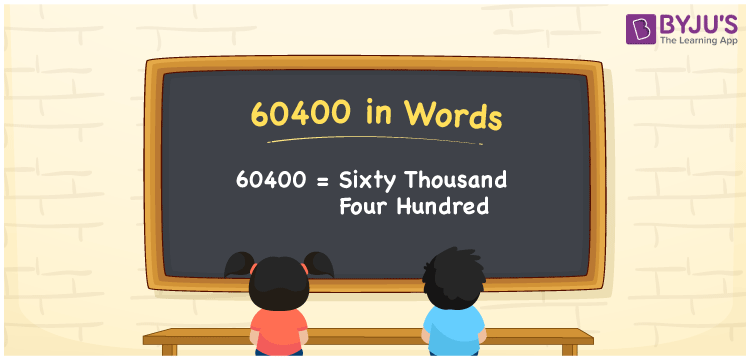# 60400 in words

60400 in words is written as Sixty Thousand Four Hundred. In 60400, 6 has a place value of ten thousand and 4 is in the place value of hundred. The article on Place Value gives more information. The number 60400 is used in expressions that relate to money, distance, length, social media views, and many more. For example, “The Odometer of my car has recorded Sixty Thousand Four Hundred kilometers.”

 60400 in words Sixty Thousand Four Hundred Sixty Thousand Four Hundred in Numbers 60400

## 60400 in English Words## How to Write 60400 in Words?

We can convert 60400 to words using a place value chart. This can be done as follows. The number 60400 has 5 digits, so let’s make a chart that shows the place value up to 5 digits.

 Ten thousand Thousands Hundreds Tens Ones 6 0 4 0 0

Thus, we can write the expanded form as:

6 × Ten thousand + 0 × Thousand + 4 × Hundred + 0 × Ten + 0 × One

= 6 × 10000 + 0 × 1000 + 4 × 100 + 0 × 10 + 0 × 1

= 60400.

= Sixty Thousand Four Hundred.

60400 is the natural number that is succeeded by 60399 and preceded by 60401.

60400 in words – Sixty Thousand Four Hundred.

Is 60400 an odd number? – No.

Is 60400 an even number? – Yes.

Is 60400 a perfect square number? – No.

Is 60400 a perfect cube number? – No.

Is 60400 a prime number? – No.

Is 60400 a composite number? – Yes.

## Solved Example

1. Write the number 60400 in expanded form

Solution: 6 x 10000 + 0 x 1000 + 4 x 100 + 0 x 10 + 0 x 1

Or Just 6 x 10000 + 4 x 100

We can write 60400 = 40000 + 0000 + 400 + 0 + 0

= 6 x 10000 + 0 x 1000 + 4 x 100 + 0 x 10 + 0 x 1.

## Frequently Asked Questions on 60400 in words

Q1

### How to write the number 60400 in words?

60400 in words is written as Sixty Thousand Four Hundred.
Q2

### Is 60400 divisible by 3?

No. 60400 is not divisible by 3.
Q3

### Is 60400 a prime number?

No. 60400 is not a prime number.